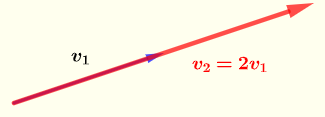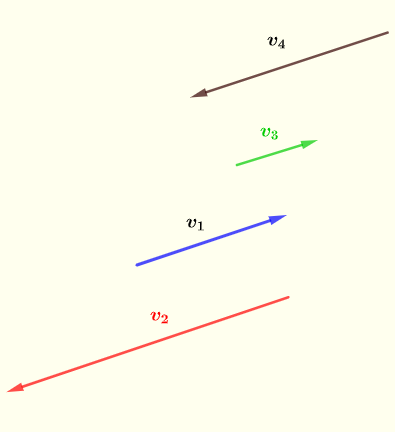# Testing for Linearity of Vectors in a Subspace - Examples with Solutions

  

Given a set of vectors in a subspace, how do we test whether the vectors are linearly independent ?

## What are Linearly Dependent or Independent Vectors?

We first use vectors in two and three dimensional spaces to visualize the concept of dependence and independence of vectors.
In figure 1 below we have have two vectors that are parallel such that $v_2 = 2 v_1$. We say that these two vectors are dependent because we can express one vector in terms of the other as follows:
$v_2 = 2 v_1$ or $v_1 = \dfrac{1}{2} v_1$.Fig.1 - Vectors $v_1$ and $v_2$ are dependent because they are parallel
In figure 2 below we have have two vectors that are parallel such that $v_2 = - v_1$. These two vectors are dependent because we can express one vector in terms of the other.Fig.2 - Vectors $v_1$ and $v_2$ are dependent because they are parallel
In figure 3, all vectors are parallel to each other. All These vectors are dependent because we can express one vector in terms of the other(s).Fig.3 - Vectors $v_1$, $v_2$, $v_3$ and $v_4$ are dependent because they are parallel
In figure 4, we can never express one vector in terms of the other because they are not parallel. These vectors are independent because we cannot express one vector in terms of the other.Fig.4 - Vectors $v_1$ and $v_2$ are independent because they are not parallel
In figure 5, using the geometrical sums of vectors, we can write
$3 v_4 = 2 v_1 + 3 v_2 + v_3$
and therefore these vectors are linearly dependent because we can express one vector in terms of the others.
In figure 6, the 3 vectors $v_1$ , $v_2 \}$ and $v_3$ are in the same plane $P$ and are therefore dependent because we can express any of these vectors in term of the other two vectors using linear combinations.
In figure 7, the pairs of vectors $\{v_1 , v_2\}$ , $\{v_2 , v_3\}$ and $\{v_1 , v_3\}$ are in different planes and are therefore independent because we cannot express any of these vectors in term of the other two vectors using linear combinations.

## Formal Definiton of Linearity of Vectors

Vectors $v_1, v_2 .... v_n$ are linearly dependent when at least one of the vectors may be expressed as a linear combinations of the remaining vectors as follows
$v_1 = r_2 v_2 + r_3 v_3 + .... + r_n v_n$
Writing the above with the zero on the right side, we obtain
$v_1 - r_2 v_2 - r_3 v_3 - .... - r_n v_n = 0$
Hence the following definition
Given a set of vectors $S = \{\textbf{v}_1 , \textbf{v}_2, ... , \textbf{v}_n \}$ ,
If the equation
$r_1 \textbf{v}_1 + r_2 \textbf{v}_2 + ... + r_n\textbf{v}_n = \textbf{0}$         (I)
has only one trivial solution $r_1 = 0 , r_2 = 0 , ... , r_n = 0$, we say that $S$ is a set of linearly independent vectors.
If the above equation has other solutions where not all $r_i$ equal to zero, then $S$ is a set of linearly dependent vectors.
In the examples below, matrices are row reduced in order to test for linearity. This may done using the row reduce augmented matrices calculator included.

## Examples with Solutions

Example 1
Are the vectors in the set $\left \{ \begin{bmatrix} -2 \\ 1 \end {bmatrix} , \begin{bmatrix} 6 \\ -3 \end {bmatrix} \right \}$ linearly independent?

Solution to Example 1
Let $v_1 = \begin{bmatrix} -2 \\ 1 \end {bmatrix}$ and $v_2 = \begin{bmatrix} 6 \\ -3 \end {bmatrix}$
The question could be answered by noticing that $v_2 = - 3 v_1$ and therefore the given vectors $v_1$ and $v_2$ are dependent (not independent)
The above was possible because we are dealing with vectors of small dimension. (2 components only)

We will now use a method that may be applied in any situation.
According to the definition given above, we need to find $r_1$ and $r_2$ such that
$r_1 v_1 + r_2 v_2 = 0$
The above may be written in matrix form as follows
$[ v_1 \;\; v_2] \begin{bmatrix} r_1 \\ r_2 \end {bmatrix} = 0$
where $[ v_1 \;\; v_2]$ is a matrix whose columns are $v_1$ and $v_2$

Write the system of equations in augmented matrix form
$\begin{bmatrix} -2 &6&|&0\\ 1 & -3&|&0 \end{bmatrix}$
Use the Gauss Jordan method to row reduce the above augmented matrix and obtain
$\begin{bmatrix} 1 &-3&|&0\\ 0 & 0&|&0 \end{bmatrix}$       (I)
The solution to the above reduced system (which is also a solution to the system before reduction) is found as follows
The second equation gives: $0 r_2 = 0$ therefore $r_2$ may take any real value
The first equation gives: $r_1 = 3 r_2$
The solution set may be written as: $\left \{ \begin{bmatrix} 3 r_2\\ r_2 \end{bmatrix} \right \} , r_2 \in R$
which therefore means that we have an infinite number of solutions and therefore the two vectors are linearly dependent.

Note that you do not have to solve the system in order to decide whether the given vectors are dependent or independent.
Construct the augmented matrix using the vectors as columns of the matrix and the constant column on the right is all zeros which in fact may be omitted. We then row reduce the augmented matrix. Then the following conclusions may easily be drawn:
1) The columns with a pivot are independent of each other
2) The columns with no pivots are dependent on the ones with the pivot.
3) The coefficients in the columns without pivot gives the coefficients of dependence on the independent columns.

Let us apply the above to the reduced matrix (I) above
1) Column 1 has a pivot and may be considered as independent
2) Column 2 has no pivot and may therefore be considered as dependent on column (1)
3) The coefficient $- 3$ in column 2 is telling us that $v_2 = - 3 v_1$

Example 2
Are the vectors in the set $\left \{ \begin{bmatrix} -2 \\ 1 \\ 4 \end {bmatrix} , \begin{bmatrix} 1\\ 0 \\ 5 \end {bmatrix} , \begin{bmatrix} 1\\ 2 \\ -1 \end {bmatrix} \right \}$ linearly independent?

Solution to Example 2
step 1: Construct the augmented matrix
Construct the augmented matrix whose columns are the given vectors and zeros on the right column
$\begin{bmatrix} -2 &1&1&|&0\\ 1 & 0&2&|&0 \\ 4 & 5 & -1& |& 0 \end{bmatrix}$

step 2: Row reduce the above matrix (you may use the row reduce augmented matrices calculator included).
$\begin{bmatrix} 1 &0&0&|&0\\ 0 & 1&0&|&0 \\ 0 & 0 & 1& |& 0 \end{bmatrix}$

step 3: Draw conclusions from the row reduced matrix
All 3 columns have a pivot each and therefore all 3 given vectors are independent

Example 3
Are the vectors in the set $\left \{ \begin{bmatrix} 2 \\ -1 \\ 3\\ 1 \end {bmatrix} , \begin{bmatrix} 0 \\ 2 \\ -1\\ 1 \end {bmatrix} , \begin{bmatrix} 4 \\ -8 \\ 9\\ -1 \end {bmatrix} \right \}$ linearly independent?

Solution to Example 3
step 1: Construct the augmented matrix using the given vectors as columns and zeros on the right column
$\begin{bmatrix} 2&0&4&|&0\\ -1&2&-8&|&0\\ 3&-1&9&|&0\\ 1&1&-1&|&0 \end{bmatrix}$

step 2: Row reduce the above matrix
$\begin{bmatrix} 1&0&\color{red}{2}&|&0\\ 0&1&\color{blue}{-3}&|&0\\ 0&0&0&|&0\\ 0&0&0&|&0 \end{bmatrix}$

step 3: Draw conclusions
Only the first and second columns (from the left) have a pivot and therefore the given vectors are not independent.
The coefficients $\color{red}{2}$ and $\color{blue}{-3}$ in the third column give the dependence of the original third columns as a linear combination of the other first and the second columns as follows
$\begin{bmatrix} 4 \\ -8 \\ 9\\ -1 \end {bmatrix} = \color{red}{2} \begin{bmatrix} 2 \\ -1 \\ 3\\ 1 \end {bmatrix} - \color{blue}{3} \begin{bmatrix} 0 \\ 2 \\ -1\\ 1 \end {bmatrix}$